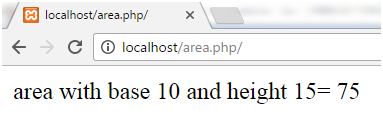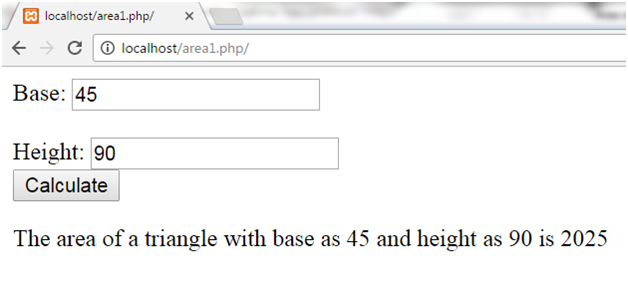Q:

# Area of Triangle in Php

Area of a triangle is calculated by the following Mathematical formula,

(base * height) / 2 = Area

## Area of Triangle in PHP

Program to calculate area of triangle with base as 10 and height as 15 is shown.

Example:

``````<?php
\$base = 10;
\$height = 15;
echo "area with base \$base and height \$height= " . (\$base * \$height) / 2;
?>  ``````

Output:## Area of Triangle with Form in PHP

Program to calculate area of triangle by inserting values in the form is shown.

Example:

``````<html>
<body>
<form method = "post">
Base: <input type="number" name="base">
<br><br>
Height: <input type="number" name="height"><br>
<input type = "submit" name = "submit" value="Calculate">
</form>
</body>
</html>
<?php
if(isset(\$_POST['submit']))
{
\$base = \$_POST['base'];
\$height = \$_POST['height'];
\$area = (\$base*\$height) / 2;
echo "The area of a triangle with base as \$base and height as \$height is \$area";
}
?>   ``````

Output: